# Calculated fields, level-aware aggregations, and evaluation order in Amazon QuickSight

Amazon QuickSight is a fast, cloud-native, serverless, business intelligence service that makes it easy to deliver insights to everyone. QuickSight has carefully designed concepts and features that enable analysis builders, such as QuickSight authors, to design content-rich, interactive, and dynamic dashboards to share with dashboard viewers. As authors build an analysis, QuickSight transforms, filters, and aggregates data from tabular datasets into result sets to answer business questions. You can implement sophisticated data analytics in QuickSight in minutes by using calculated fields, then share within QuickSight in your organization, or embedded into apps or portals to share with thousands of users without any servers or infrastructure to set up.

This post gives you an end-to-end overview of how to perform various calculations in QuickSight and introduces you to the concepts of evaluation order and level-aware aggregation, which allow you to build more advanced analytics that use scalar, aggregate, and table functions. We also explain these approaches using an analogy to SQL.

This post assumes that you have a basic knowledge of analytics, SQL, and QuickSight.

## Sample dataset and the business question

For this post, we use the Patient-Info dataset, which holds fictional transactional records for inpatient services. It contains dummy data that is randomly generated by AWS for demonstration purposes. The tabular table has the following columns:

• Patient ID – ID of the patient
• Hospital – Name of the hospital
• Service – Service item provided during inpatient visit
• Category – Category of the service during inpatient visit
• Subcategory – Subcategory of the service during inpatient visit
• Revenue – Revenue from the service rendered
• Profit – Profit from the service rendered

For instructions on creating a SPICE dataset in QuickSight with this dataset, see Prepare Data.

We use QuickSight to answer the following business question and variations of it from the dataset: What is the average profit ratio across all categories?

This question has a two-step calculation logic, which is common in use cases like goal completion analysis:

1. Find the profit ratio per category.
2. Find the average profit ratio across category.

In the process of answering this, we explore potential solutions in different approaches while discussing different features QuickSight has to offer:

• Scalar functions – Return a single value computed for every row of input data, such as `Plus`, `Division`
• Aggregation functions – Operate against a collection of values and return a single summarized value, such as `Avg()`
• Table functions – Operate against a collection of rows and return a collection of rows, such as `Rank()`, `avgOver()`, `sumOver()`
• Level-aware aggregation – A special type of table function that is evaluated before aggregation or before filtering

Some of these potential solutions don’t lead to the desired answer. But you will have a deep understanding of these QuickSight function types by thinking about why they don’t work. You can also jump to the definition of the calculated field `Average Profit Ratio M` to see the final solution.

## Scalar functions

After the SPICE dataset is created with `Patient-Info`, let’s create an analysis from the dataset, and then try to find the answer to the business question using scalar functions.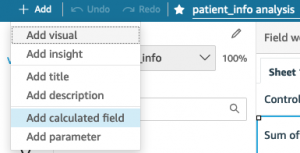1. In the calculated field editor, enter the name and formula:
``Profit Ratio = profit / revenue``
1. Choose Save.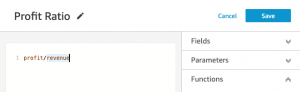1. Add `Profit Ratio` to a KPI visual. Remember to set the aggregate function to Average because we want to find the average profit ratio.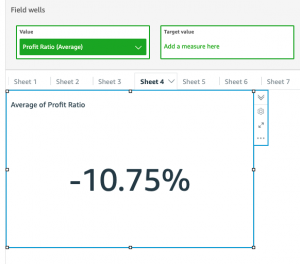1. Add `category` and `Profit Ratio` to a table visual. Again, we want to set the aggregate function to `Average`.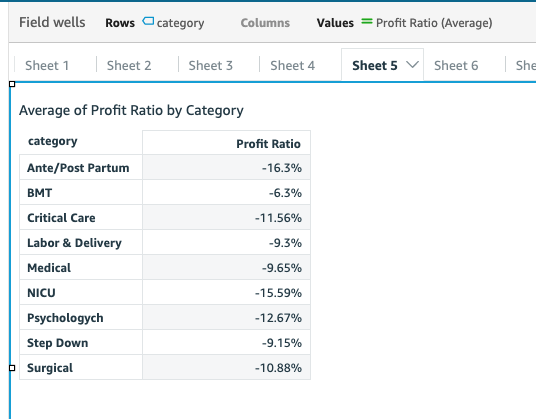What is calculated here? Our dataset is at transactional level, so QuickSight calculates the profit ratio for every transaction and aggregates the results to the desired level defined in Visuals.

The calculation QuickSight has performed is similar to the following code:

``````select avg(profit/revenue)
from dataset                -- to calculate the KPI visual

select category, avg(profit/revenue)
from dataset
group by category                -- to calculate the table visual``````

This isn’t the answer we’re looking for because the profit ratio for a category is defined as the total profit of the category divided by the total revenue of the category.

## Aggregate functions

Let’s try a different approach using aggregate functions:

``Profit Ratio with Agg Func = sum(profit)/sum(revenue)``

QuickSight is smart enough to figure out that author wants to aggregate data to the visual level first, and then use the division.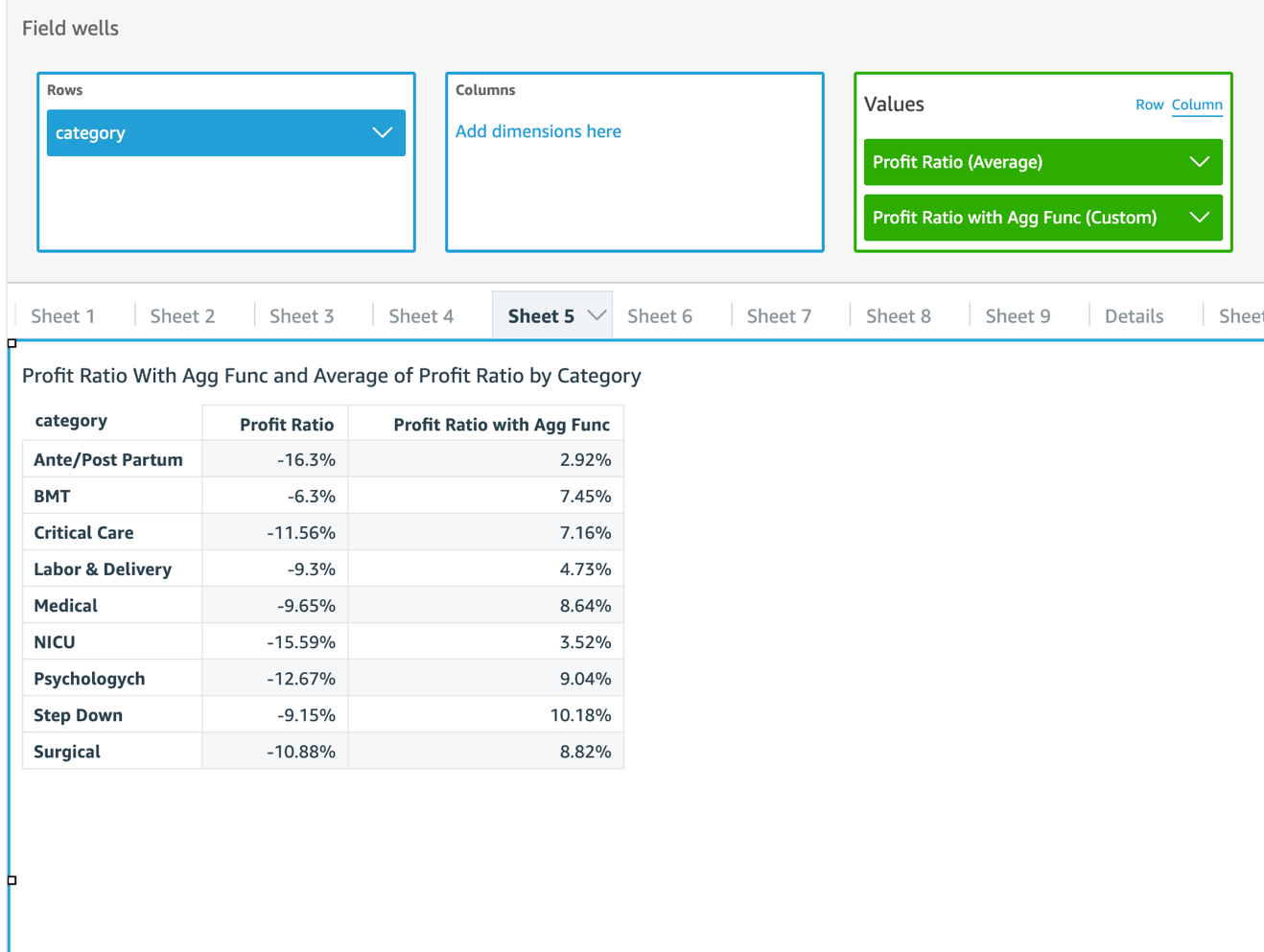When we compare the results with `Profit Ratio` we created earlier, the numbers are quite different! This is because `Profit Ratio` calculates the transactional-level ratio first and then finds the average; whereas `Profit Ratio with Agg Func` calculates the category-level totals of the numerator and denominator first and then finds the ratio. Therefore, `Profit Ratio` is skewed by some big percentage loss in certain transactions, whereas `Profit Ratio with Agg Func` returns more meaningful data.

The calculation can be modeled in SQL as the following:

``````select category
, avg(profit/revenue) as "Profit Ratio"
, sum(profit)/sum(revenue) as "Profit Ratio with Agg Func"
from dataset
group by category   ``````

`Profit Ratio with Agg Func` returns the category-level profit ratio we wanted. The next step is to find an average of the ratios.

## Table functions

Now let’s look for help from table functions. A table function outputs the same number of rows as input, and by default it has to be used on top of another aggregation function. To find the average of profit ratios, we can try `avgOver`():

``avgOver of Profit Ratio = avgOver({Profit Ratio with Agg Func})``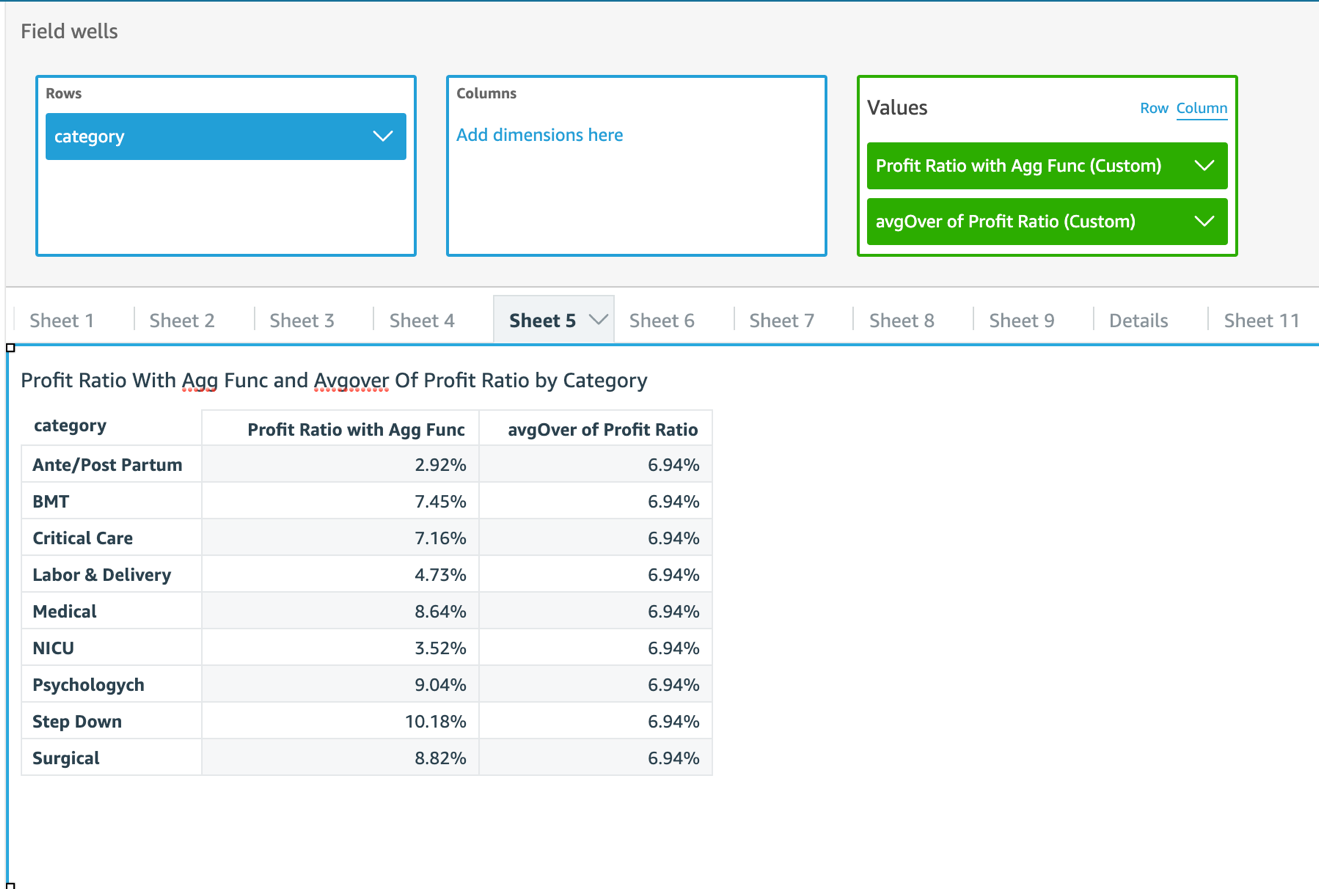The following code is the corresponding SQL:

``````with aggregation_step as (
select category
, sum(profit)/sum(revenue) as "Profit Ratio with Agg Func"
from dataset
group by category
),
select category
, "Profit Ratio with Agg Func"
, avg("Profit Ratio with Agg Func") over()
as "avgOver of Profit Ratio"
from aggregation_step``````

This example is complicated enough that QuickSight has to follow a sequence of steps to calculate a single visual. By default, QuickSight goes through up to six stages to complete the calculations for a visual:

1. Simple calculations – Scalar calculations that can be applied before filter and aggregation
2. Analysis filters – Apply filters on dimensions and measures with no aggregation option selected
3. Top/bottom N filters – A special type of filter that is defined on a dimension, and sorted by a field that doesn’t contain table functions
4. ON-VISUAL – Aggregations (evaluate `group by` and aggregations) and filters (apply filters with aggregation in the `having` clause)
5. Table calculations – Calculate table functions and evaluate filters with table functions
6. Totals and subtotals – Calculate totals and subtotals

With `avgOver()`, we’ve got the answer we’re looking for: 6.94%. However, the number is displayed for every category, which is not preferred. Actually, we can only get this number when the category is on the visual.

When the category is removed, `Profit Ratio with Agg Func` is aggregated to the grand total level in the aggregation step, therefore its `avgOver` remains the same number, as shown in the following screenshot.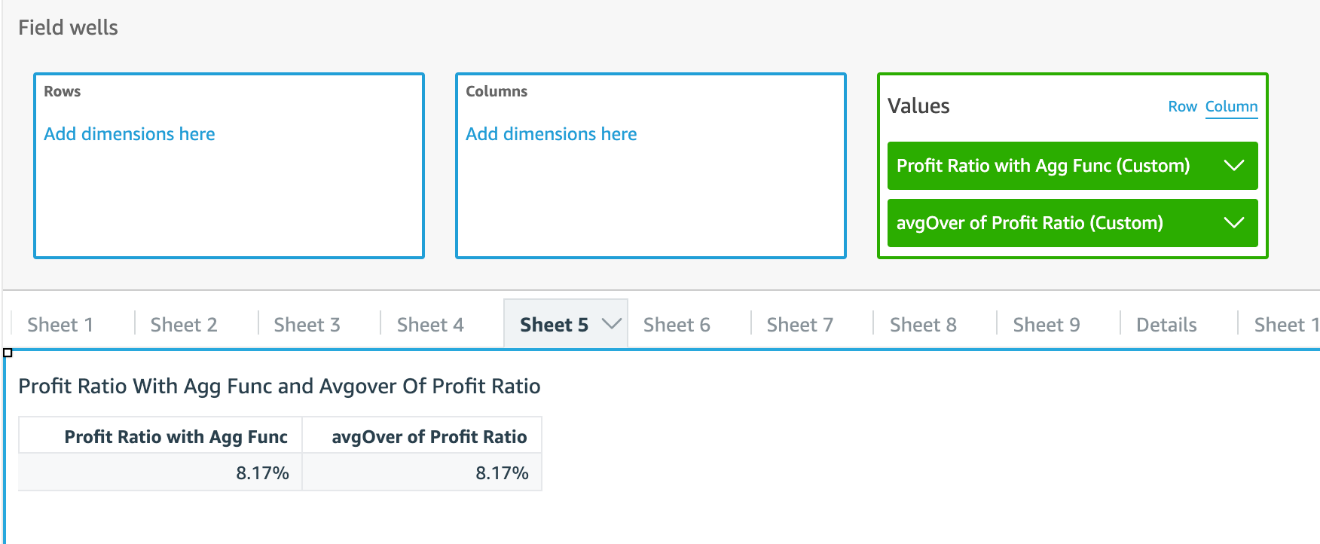To avoid these drawbacks, we need a new tool.

## Level-aware aggregations

QuickSight introduced a type of calculation mechanism called level-aware aggregation (LAA) to meet more analytical requirements. Like table functions, LAAs operate against a collection of rows and return the same number of rows. Regular table functions can only be evaluated after the aggregation and filter stage in QuickSight. With LAA, authors can evaluate a group of functions before aggregations or even before filters.

The following diagram illustrates the evaluation order of LAA.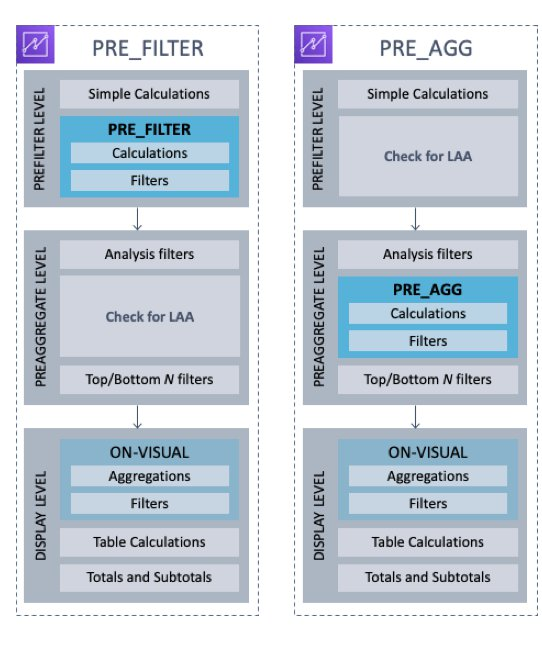Because LAA is evaluated before aggregation, both its input and output are at the dataset level. Calculated fields with LAA behave similarly to calculated fields with scalar functions. It can be specified as a dimension or a measure. An aggregation function needs to be applied on top of LAA when the calculated field is used as a measure in visuals. When you want to filter on a calculated filed with LAA, QuickSight asks you to choose between no aggregation or one aggregation function. Also, duplicated rows are likely populated within the partition groups because the output level of LAA remains at the dataset level.

It seems that we can use `sumOver` with `category` as the partition group, and then use average as the aggregate function to find the answer:

``````sumOver(profit) = sumOver(profit,[category],PRE_AGG)
sumOver(revenue) = sumOver(reveune,[category],PRE_AGG)
countOver(profit) = countOver(profit,[category],PRE_AGG)

Average Profit Ratio = avg({sumOver(profit)}/{sumOver(revenue)})``````

The following screenshot shows the aggregation functions defined for each measure. `countOver(profit)`with `min()` as aggregate simply returns transaction counts per category. It’s also the number of duplicated rows `sumOver(profit)` and `sumOver(revenue)` output.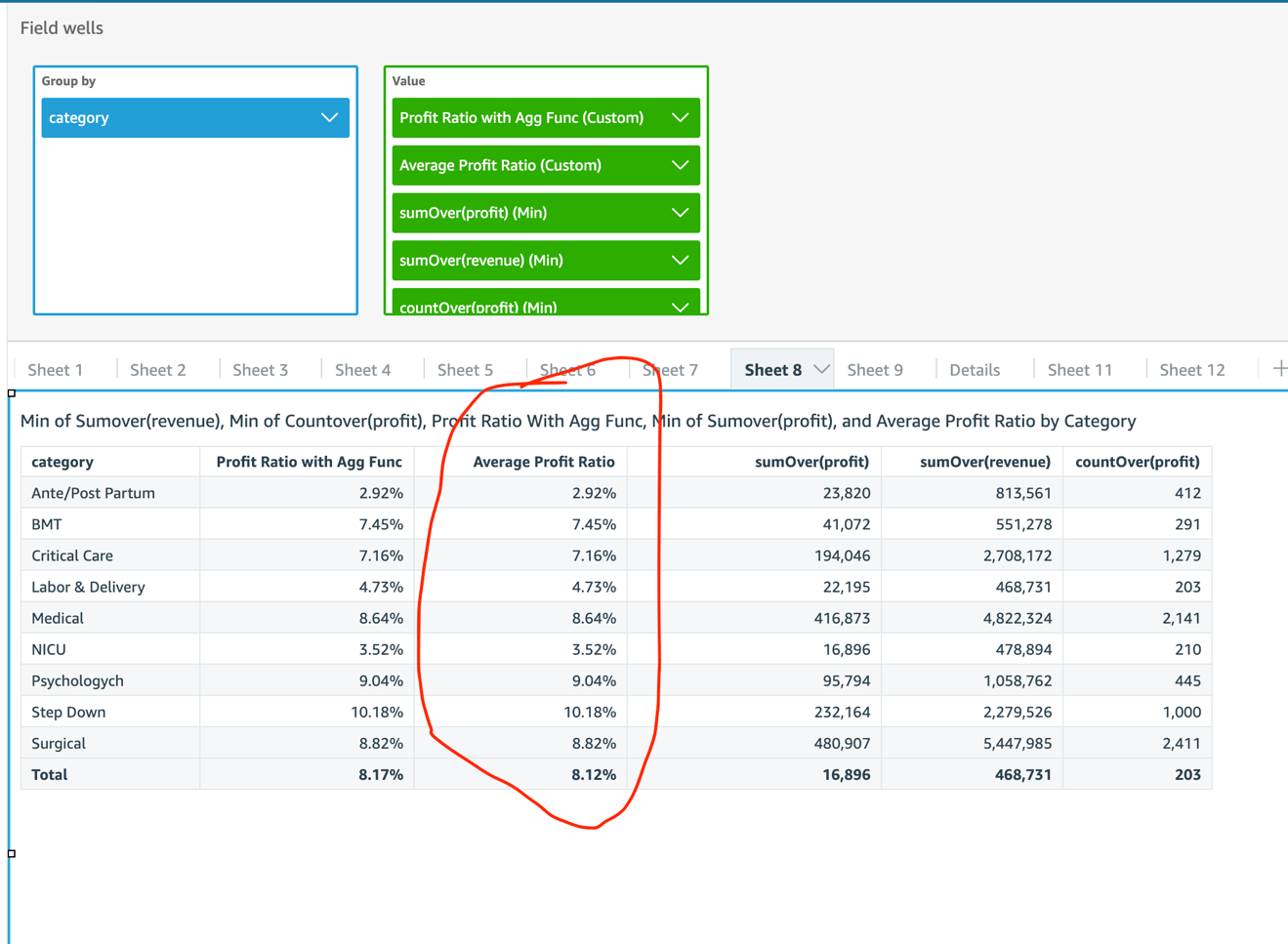8.12% is not the correct answer to the business question. The correct average should be 6.94%, as we saw earlier. How does QuickSight come up with the number?

For `Average Profit Ratio`, QuickSight tried to calculate the following:

``````with LAA as (
select category
, sum(profit) over (partition by category) as "sumOver(profit)"
, sum(revenue) over (partition by category) as "sumOver(revenue)"
, count(profit) over (partition by category) as "countOver(profit)"
from dataset
),                       -- notice that LAA is at the same level of dataset

select category,
, avg("sumOver(profit)" / "sumOver(revenue)") as "Average Profit Ratio"
from LAA
group by category;       -- for data at category level

select avg("sumOver(profit)" / "sumOver(revenue)") as "Average Profit Ratio"
from LAA;                --  for data at total level``````

This is a smart approach. But each category has a different number of transactions, therefore each category-level `Profit Ratio` has a different number of duplicated rows. The average in the last step is equivalent to a weighted average of category-level `Profit Ratio`—weighted by the number of duplicates.

We want to modify `Average Profit Ratio` to offset the weights. We start with the following formula:

``Average Profit Ratio M = Sum(Profit Ratio per Category)/number of Categories``

We know the following:

``````Profit Ratio from LAA = sumOver(profit) / sumOver(revenue)
number of Categories = distinct_count(category)``````

How can we handle the duplicated rows? We can divide `Profit Ratio` by the number of duplicates before summing them up:

``````Sum(Profit Ratio per Category) = Sum(Profit Ratio from LAA / number of duplicate rows per Category)

number of duplicate rows per Category = countOver(profit)``````

Put them together, and we can create the following:

``Average Profit Ratio M = sum( sumOver(profit) / sumOver(revenue) / countOver(profit) ) / distinct_count(category)``

In this dataset, `countOver(profit)` are large numbers in which intermediate results may be dimmed to zero because they’re smaller than QuickSight’s precision, so we can add another factor 10000 to inflate intermediate results and deflate the final output:

``Average Profit Ratio M = sum( 10000 * sumOver(profit) / sumOver(revenue) / countOver(profit) ) / distinct_count(category) / 10000``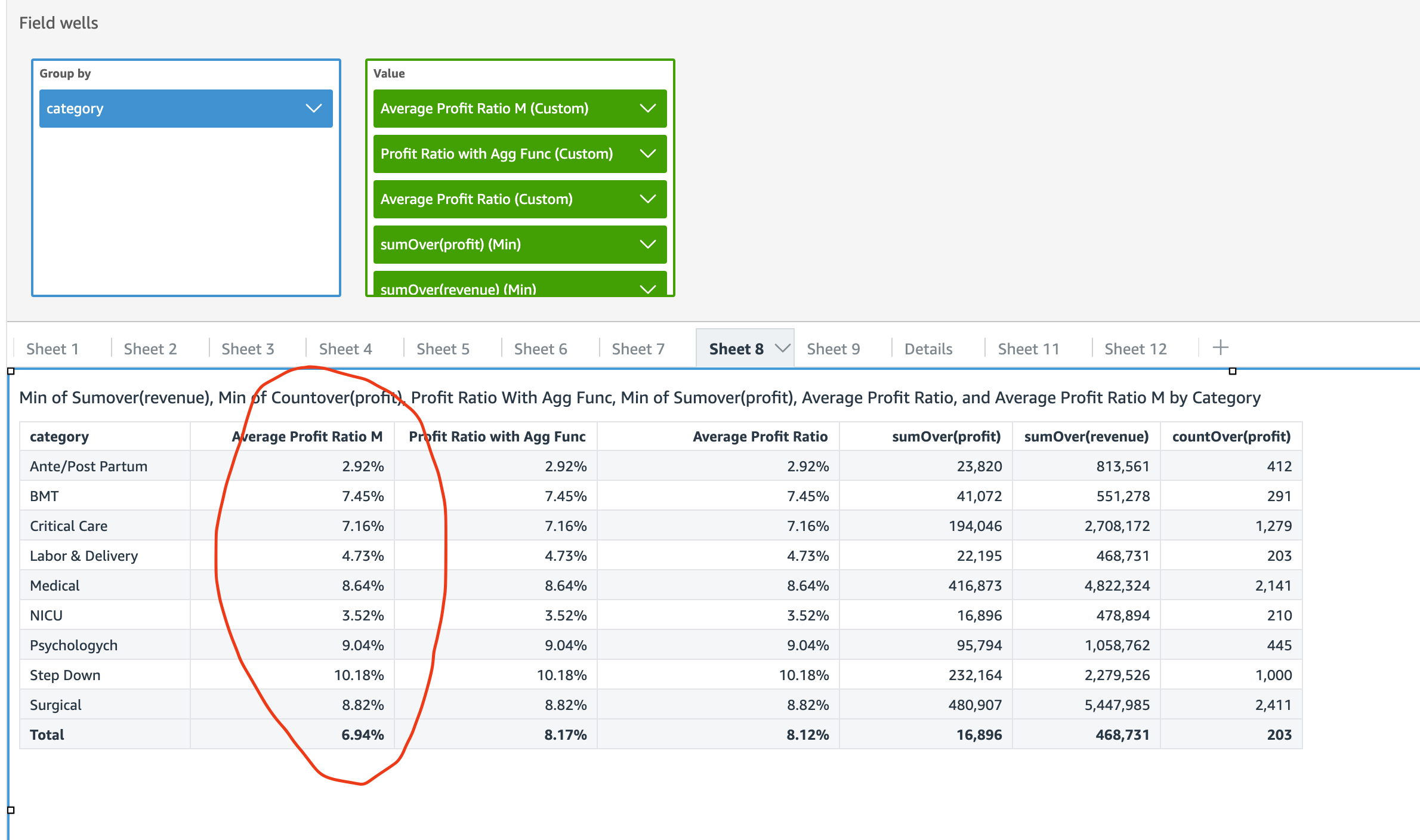6.94% in total is what is expected!

For `Average Profit Ratio M`, QuickSight tried to calculate in the following steps:

``````with LAA as (
select category
, sum(profit) over (partition by category) as "sumOver(profit)"
, sum(revenue) over (partition by category) as "sumOver(revenue)"
, count(profit) over (partition by category) as "countOver(profit)"
from dataset
),                       -- notice that LAA is at the same level of dataset

select category,
, sum(10000 * "sumOver(profit)" / "sumOver(revenue)" / "countOver(profit)")
/ count(distinct category) / 10000 as "Average Profit Ratio"
from LAA
group by category;       -- for data at category level

select sum(10000 * "sumOver(profit)" / "sumOver(revenue)" / "countOver(profit)")
/ count(distinct category) / 10000 as "Average Profit Ratio"
from LAA;                -- for data at total level``````

## Conclusion

This post discussed how you can build powerful and complicated data analytics using QuickSight. We also used SQL-like scripts to help you better understand QuickSight concepts and features.Ian Liao is a Data Visualization Engineer with the Data & Analytics Global Specialty Practice in AWS Professional Services.